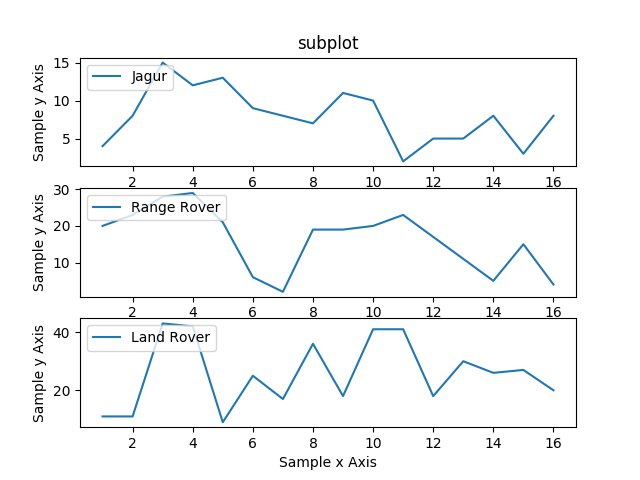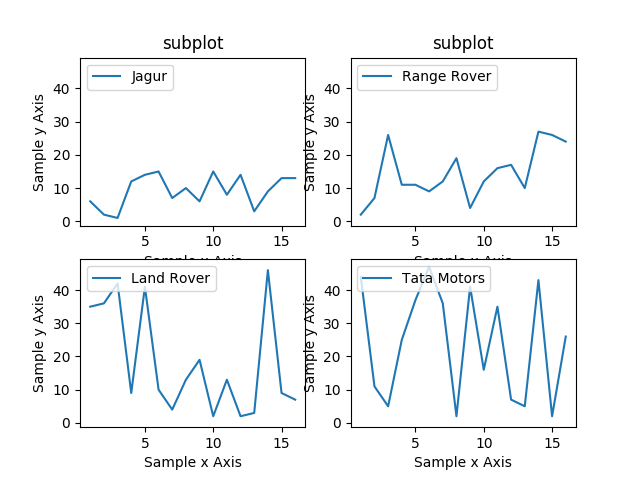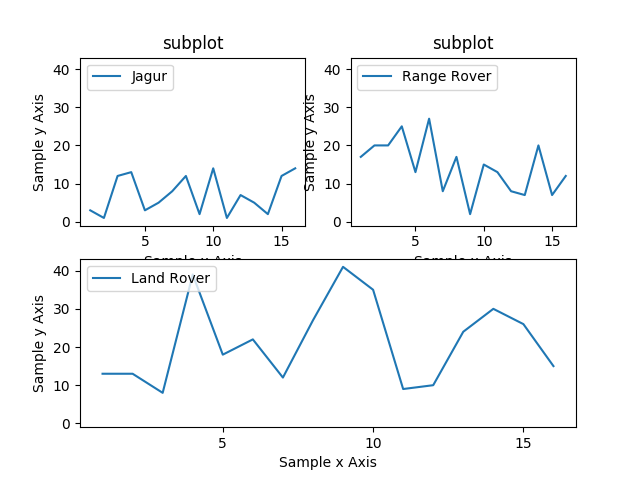# How to use Subplots in matplotlib Data Visualization using Python

Many a times, I needed multiple plots in the same view as it helps in analyzing data in proper way. Also, if you want to present this data somewhere, it helps to plot two graphs together.

One alternative to subplots is using multiple axis and plotting 2 data points on the same graph but this might distort the view. Each has its own applications. In this article, we will look at how to plot  subplots.

Sytax is as below

`subplot(nrows ncols index)`

Here, nrows and ncols need not be same for all plots. Its only specific to current plot. Please refer to third example in this article

• Plotting three graphs using matplotlib

Output is as below• Plotting 4 graphs in 2*2 grid using matplotlibb

Output is as below• Plotting 3 graphs using matplotlib